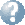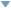Online Reference Dictionary, Encyclopedia & moreby: WordStarts with Ends withMentionsteaching:topics:algebra:first

Number Algebra is the symbolic language that we use to write down and work with ‘facts’ we are given, or ‘facts’ we want to know, about numbers and things we can measure or count that behave like numbers.

We use letters to represent the values we are interested in but do not know yet, the ‘unknowns’ in our problem, the values we are trying to find.

We write algebra a little differently compared to arithmetic. We do not usually write the times or div symbols. We always use a fraction-bar instead of div and we write numbers and letters next to each other to mean multiplication, so that: \begin{align*} \phantom{\frac12}42ab\ \ \text{means}\ \ &42 \times a \times b,\\ \text{and:}\ \ \ &\\ \frac{4x}{yz}\ \ \text{means}\ \ &(4 \times x)\div(y \times z). \end{align*} We call these groups of symbols a term.

An expression describes a sequence of operations on letters and numbers. Brackets around an expression make it a sub-expression. A sub-expression has a value, it fits anywhere a letter would fit. A fraction-bar also acts like brackets, the numerator, denominator and the algebraic fraction itself are each sub expressions. An expression can be a sum or difference of terms and sub-expressions. An example: $3x^2 + \frac{x^2-4}{x-2} - 3(2y-3)^3.$ It includes four sub-expressions: $\quad x^2-4;\ \ x-2;\ \ \frac{x^2-4}{x-2};\ \ 2y-3.$

An equation uses an $=,$ it is a statement that two expressions have the same numerical value. It is often a description of a ‘fact’ we know about the unknown quantities we are trying to find. In all of this each letter always represents the same quantity, we use different letters when we want to talk about different quantities. Uppercase and lowercase letters are distinct. This all starts out very much like arithmetic, but with some numbers we do not know yet. But we are mathematicians, so we have abstracted and generalised and made it very much more powerful than simple arithmetic. A simple but not trivial arithmetic-like example: $\large 2a - 3(b+c) = a-2c.$

Working with number means using the rules that apply to all the things we consider to act like numbers — including measurements of lengths, time, angles, areas, volumes and mass, also probabilities and many other quantities.

After writing down the facts we know about the values we want to find we then play around with them. We rewrite them in different ways and combine them in different ways — finding related facts about these unknown values — often seeking some equation, some fact, that involves only one of our unknowns. Then we rewrite this equation until it looks like $x=\texttt{number},\quad$ that is: we find the equivalent equation-fact that tells us the value of that unknown. Then we use that value and look for the value of another — we are closer to a solution, our problem has become one unknown simpler.

Early in learning algebra we look at problems that we could solve without writing them down like this, but real problems in the world, and even the simplified problems we soon look at, are too complex to hold in your head as a single puzzle to solve. (Or perhaps your teacher is not giving you problems that are challenging enough!) We need to break them down into parts and keep track of what we have found along the way. To do that we need to write it all down very systematically. We need a formal language to express problems in, and a language we have built for that is algebra.